# Experimental Performance and Economic Evaluation of a Thermoelectric Liquefied Petroleum Gas (TE-LPG) Cook Stove

Experimental Performance and Economic Evaluation of a Thermoelectric Liquefied Petroleum Gas (TE-LPG) Cook Stove

Charoenporn LertsatitthanakornPawatwong Bamroongkhan Kitti Sathapornprasath Somchart Soponronnarit

Energy Management Technology Division, School of Energy, Environment and Materials, King Mongkut’s University of Technology Thonburi, Bangkok, 10140, Thailand

Energy Technology Division, School of Energy, Environment and Materials, King Mongkut’s University of Technology Thonburi, Bangkok, 10140, Thailand

Department of Mechanical Engineering, Faculty of Engineering, Srinakharinwirot University, 63, Moo 7, Ongkharak, Nakorn Nayok, 26120, Thailand

Corresponding Author Email:
charoenporn.ler@kmutt.ac.th
Page:
575-582
|
DOI:
https://doi.org/10.18280/ijht.370227
23 January 2018
|
Accepted:
29 April 2019
|
Published:
30 June 2019
| Citation

OPEN ACCESS

Abstract:

The use of liquefied petroleum gas (LPG) cook stoves is widespread in households throughout Thailand. The LPG is burned by a cooker-top burner that gives off high thermal energy, some of which is lost to the environment. This lost heat can be used to drive thermoelectric (TE) modules which in turn produce electrical power. The TE-LPG cook stove comprises TE power modules, a dual purpose wind shield used to protect against wind flowing to the cooker-top burner while simultaneously serving as the hot side of the TE modules, a fin heat sink at the cold side of the TE modules, and a fan. An experimental set-up was built to evaluate the conversion efficiency at various temperature ranges. The electricity produced was used to charge a lead-acid battery that drove a fan and some auxiliary features. The results showed that a maximum power output of 9.3 W was obtained. A theoretical model was then developed to describe the experimental results taking in to account convection and conduction phenomena at the cold side heat sink. An economic analysis indicates that payback period of the TE power generation system depends on the annual operating time.

Keywords:

thermoelectric, cook stove, conversion efficiency, payback period

1. Introduction

In developing countries, energy used for cooking constitutes a significant portion of the total energy requirement. In Thailand, for example, 59 % of all LPG was consumed by the household sector . The average thermal efficiency of LPG cooker-top burners in Thailand is 50%. Therefore, the heat loss from the of LPG cooker-top burners is around 50 %. In view of such large scale usage, even heat recovery from the heat loss of LPG cook stoves will lead to meaningful importance with respect to the economy of fuel usage. One interesting method used to convert thermal energy loss into useful electrical energy is TE power generation. In past years, numerous TE generators of varying designs have been incorporated with cook stoves.

Nuwayhid et al.  studied the development and test of a prototype TE generator intended for use in rural domestic wood stoves in regions with unreliable electrical service. The stove-top TE system produced a maximum power output of 100 W by using 30 TE modules. Lertsatitthanakorn  developed and tested a biomass cook stove TE generator. In that system a TE power module was installed on a sheet metal wall which acted as one side of the stove’s structure. The TE module was cooled by a rectangular fin heat sink. The results showed that the maximum power output from the TE generator was 2.4 W at the temperature difference of approximately 150 °C. An economic evaluation indicated that the payback period tended to be very short when compared with the cost of the same power supplied by batteries. Champier et al.  designed and tested the TE generation system with a biomass stove. Four TE modules were used. A water tank was installed on the cold side of the TE modules as the cooling system. Maximum power output was 7 W for a temperature difference between the hot and cold sides of TE modules of 160 °C. Champier et al.  studied the integrating and testing of a TE generator in a multifunction biomass stove. A one dimensional TE model was developed and compared with the experimental data, which showed good agreement. The TE generator produced a power output of 9.5 W at maximum load. An economic study was adopted to compare the cost of solar cell panels with a multifunction cooking stove with the TE generator. The result showed that the cost of power from the TE module was very competitive with the solar cell panels. O’Shaughnessy et al.  investigated the integration of a TE module with a portable biomass cook stove. The results showed that the TE generated an average of 3 Wh of electrical energy during a typical 1 h burn. The generated power was enough to charge a single 3.3 V lithium-iron battery and drive a low power fan. Montecucco et al.  built a combined heat and power (CHP) system by incorporating a TE generator with a solid-fuel stove. The TE system produced 27 W of electric energy. The conversion efficiency of the TE system was around 5 %. The water cooling system was used to release heat from the cold side of TE generator, which produced 600 W of thermal energy. The temperature of the water in the storage tank was raised by 20 °C. Najjar and Kseibi  developed a multi-task TE JUST (Jordan University of Science and Technology) stove. The stove consisted of 12 TE generator modules, water heater and cooker area. The fin heat sinks were fixed at both sides of the TE modules. Three types of fuel were used in this study namely: wood, peat and manure (horses). Results indicate that the maximum power output was 10.25 W at the maximum temperature difference 43 °C by using wood as fuel. Li et al.  constructed and tested a stove-powered TE generator with self-starting fan cooling. A novel heat collector was installed in the combustion chamber for conducting the heat to the TE modules. Hard charcoal was used as fuel to testing the prototype. Experimental results showed that the TE stove can be self-startup. The maximum power output was 12 W, at the maximum temperature of 147 °C which corresponded to the TE conversion efficiency of 2.8 %. Obernberger et al.  studied a new micro CHP system based on a wood pellet stove (thermal capacity 10.5 kW) with a TE generator. 12 TE modules were incorporated with the wood stove. The maximum power output can be reached to 50 W. Lv et al.  built and tested a compact water-cooled TE generator combined with portable gas stove. A U-shaped copper heat-conducting plate tube was used to transfer the heat from the stove to the TE modules. A water-cooled heat sink with radiator was used to release heat from the TE modules. It was found that the maximum power output was 12.9 W at the TE conversion efficiency of 2.34 %. A theoretical model of TE power generation was developed to help in the design of the TE system.

This work presents a TE generator system installed on a modified wind shield of the LPG cook stove. Experimental testing of this system was conducted and discussed. A thermal resistance network model was developed to predict the cold side temperature of the TE modules. The influences of operating parameters are experimentally determined. Finally, an economic analysis was conducted to predict the payback period of the TE power generation system. The flow chart of work approach in this study is shown in Figure 1.

## 1.png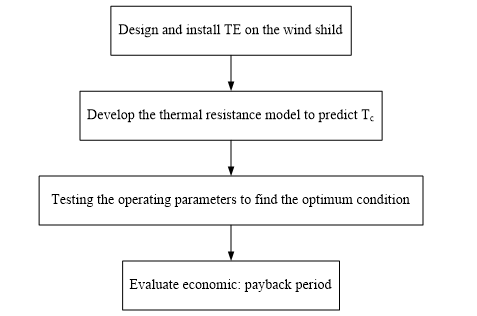Figure 1. Flow chart of the work approach in this study

2. System Description

Tests were performed on an LPG cook stove purchased from an in-country manufacturer. The cooker-top burner was self-aspirating at a relatively low supply gas pressure of not more than 280 mm H2O with corresponding input thermal power of not more than 5 kW. A radial flow burner type stove was used in this study. Thermal efficiency of the burner was approximately 41.6 %, tested by Makmool et al. . The LPG tank contained 4 kg of LPG. Originally, a curve shaped wind shield was used to protect against the wind flowing past the cooker-top burner. The curved shape interfered with the installation of the TE modules. A revised design in the shape of a plane was made and is shown in Figure 2. The newly designed wind shield was made of aluminum and installed around the cook-top burner. One side of the wind shield is a flat plane and serves as the hot side of the TE modules. A schematic view of the TE-LPG cook stove is shown in Figure 3. Meghdir et al.  suggested that forced convection air cooling achieve good performance. Therefore, a heat sink equipped with a fan was used to release from the hot side of the TE modules. The rectangular fin heat sink made of aluminum was used on the cold side. The fins were 1.3 mm thick; 85 mm long in the vertical direction and had a height of 40 mm from the base. There were 15 fins with a pitch of 3.2 mm on the base. The heat sink came equipped with a 5 V fan. The space between the TE modules, wind shield sheet and heat sink were insulated using a thermal insulator (thermal conductivity 0.039 W/mK, ). Four TE modules (model TEHP1-12635-1.2, China) made of bismuth-telluride-based alloys were used. Each module had an area of 3.5 cm × 3.5 cm. The TE modules were connected in series. Both surfaces of each TE module were coated with thermal grease to enhance heat conduction. An adjustable valve was used to control the flow rate of LPG to the cooker-top burner.

## 2.png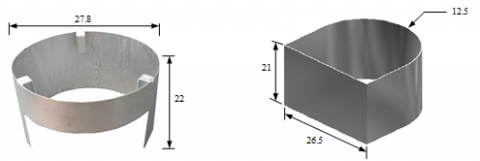(a)                                                          (b)

All dimensions are in cm.

Figure 2. Dimension of wind shield: (a) original (b) modified wind shield in this study

## 3.pngFigure 3. Schematic showing layout of TE-LPG

The electronic circuit was specifically designed to charge a battery and energize light emitting diodes (LEDs) as shown in Figure 4. The power produced by the TE system was separated into two supplies. One was used to charge a 6 V battery and the other to light the three LEDs. The battery was used to drive the fan. Thus, the fan started when the cook stove was started. The fan consumed 1 W.

## 4.pngFigure 4. Schematic of an electronic part of TE power output

Experimental tests are presented for various parameters namely, cooling air flow rate, hot side temperature with two fan orientations: (1) air being either pushed or (2) pulled through the finned area (see Figure 5).

## 5.pngFigure 5. Fan orientation

T-type (accuracy ± 0.5 °C) thermocouples were used to measure the temperature of the hot and cold sides of the TE modules, the fine heat sinks and the cooling air. Air velocity from the fan was measured by a hot bulb velocity probe (accuracy ± 0.03 m/s). A data acquisition system was used to collect the data at regular 1 minute intervals.

3. Theoretical Considerations

For this section, the electrical and thermal parts were analyzed as follow:

Electrical analysis

The electrical output of the TE modules (P) was calculated from measured data as follows:

$\mathrm{P}=\mathrm{I} \cdot \mathrm{V}$  (1)

where I and V are the electric current and voltage of the TE modules, respectively. The conversion efficiency can be evaluated as .

$\eta_{\mathrm{e}}=\eta_{\mathrm{c}} \frac{M-1}{M+\frac{T_{\mathrm{c}}}{T_{\mathrm{h}}}}$      (2)

where $M=\sqrt{1+Z T_{\mathrm{m}}}$ which $T_{\mathrm{m}}=0.5\left(T_{\mathrm{h}}+T_{\mathrm{c}}\right)$, Th and Tc are the hot and cold side temperatures of TE module, respectively.

Tm is the average temperature Z is the figure of merit of the TE material (Z=1.6 × 10-3 1/K) .

$\eta_{\mathrm{c}}$ is the Carnot efficiency;

$\eta_{\mathrm{c}}=\frac{T_{\mathrm{h}}-T_{\mathrm{c}}}{T_{\mathrm{h}}}$        (3)

Heat transfer analysis

The cold side temperature of TE module can be calculated by a thermal resistance network assuming a one-dimension heat flow from the cold side of TE module through the rectangular fin heat sink. The thermal resistance model was composed of heat sink base resistance (Rb) and convection resistance of the heat sink (Rc) as shown in Figure 6.

## 6.pngFigure 6. Thermal resistance model for TE power generation system

The heat transfer rate from the heat sink can be written as 

$\mathrm{Q}_{\mathrm{c}}=\frac{\left(\mathrm{T}_{\mathrm{c}}-\mathrm{T}_{\mathrm{a}}\right)}{\mathrm{R}_{\mathrm{t}}}$  (4)

where Ta is the ambient temperature and Rt is the total thermal resistance and is defined as:

$R_{t}=R_{c}+R_{b}$  (5)

where Rc is derived by conduction resistance model, which defined as:

$\mathrm{R}_{\mathrm{b}}=\frac{\mathrm{t}_{\mathrm{b}}}{\mathrm{k}_{\mathrm{al}} \mathrm{A}_{\mathrm{b}}}$        (6)

where Ab is the base area, kal is the thermal conductivity of aluminum and tb is the base thickness. The convection heat transfer coefficient of a rectangular fin heat sink in impingement flow is calculated by: 

$\mathrm{h}=\frac{0.49}{\left(\frac{\mathrm{L} / 2}{\mathrm{RePrD}_{\mathrm{h}}}\right)^{0.5}} \frac{\mathrm{k}_{\mathrm{a}}}{\mathrm{D}_{\mathrm{h}}}$          (7)

where ka is the thermal conductivity of air, Dh is the hydraulic diameter, which is given by:

$\mathrm{D}_{\mathrm{h}}=\frac{2 \mathrm{sH}}{\mathrm{s}+\mathrm{H}}$  (8)

where H is the height of fin and s is the fin spacing. The Reynolds number (Re) is calculated by:

$\operatorname{Re}=\frac{v_{f} D_{h}}{v}$  (9)

where $\mathbf{V}$ is the kinematic viscosity of air and vf is the air flowing over the fins, which can be calculated by knowing the air volume flow rate (V) as follows:

$\mathrm{v}_{\mathrm{f}}=\frac{\mathrm{V}}{\left(\mathrm{n}_{\mathrm{f}}-1\right) \mathrm{sH}}$  (10)

where nf is the number of fins. The convection resistance of heat sink is given by 

$\mathrm{R}_{\mathrm{c}}=\frac{1}{\mathrm{h}\left[\left(\mathrm{n}_{\mathrm{f}} 2 \mathrm{LH} \eta_{\mathrm{f}}\right)+\left(\mathrm{n}_{\mathrm{f}}-1\right) \mathrm{sL}\right]}$                  (11)

where nf is the fin efficiency is obtained by 

$\eta_{\mathrm{f}}=\frac{\tanh \left(\mathrm{H} \sqrt{\left(2 \mathrm{h} / \mathrm{k}_{\mathrm{al}} \mathrm{t}_{\mathrm{f}}\right)}\right)}{\mathrm{H} \sqrt{\left(2 \mathrm{h} / \mathrm{k}_{\mathrm{al}} \mathrm{t}_{\mathrm{f}}\right)}}$        (12)

The heat released at the cold side of the TE modules is also given by

$\mathrm{Q}_{\mathrm{c}}=\mathrm{m} \mathrm{C}_{\mathrm{pa}}\left(\mathrm{T}_{\mathrm{ao}}-\mathrm{T}_{\mathrm{ai}}\right)$  (13)

where m is the mass flow rate of the air and Cpa is the specific heat of air From Eq. (4), the cold side temperature of TE modules is given by

$\mathrm{T}_{\mathrm{c}}=\mathrm{Q}_{\mathrm{c}} \mathrm{R}_{\mathrm{t}}+\mathrm{T}_{\mathrm{a}}$  (14)

4. Results

In Figure 7, typical temperature distributions are shown for ambient air, outlet air from the heat sink, hot and cold side of the TE modules. The hot side temperature increased from 30.2 to 130.7 °C. The maximum cold side temperature was 71.8 °C. The system reached the steady state after 6 minutes operating time. The maximum temperature difference between the hot and cold side of the TE modules was 58.9 °C.

## 7.png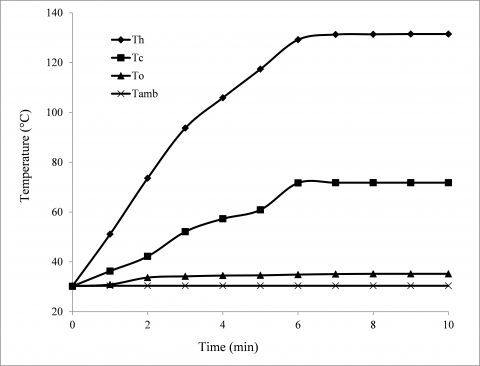Figure 7. Temperature profile of ambient air, outlet air, hot and cold sides of TE modules (hot side temperature: 130 °C and airflow rate 0.028 kg/s)

4.1 The effect of cooling air flow rate

To realize the influence of flow rate of coolant on the performance of the TE modules, the temporal distributions of temperature of the cold side at the air flow rate of 0.018, 0.025, 0.028 kg/s are presented in Figure 8, where the hot side temperature was fixed at 130 °C. It can be seen that the cold side temperature decreased, when the air flow rate increased, as more heat was rejected to the air. This is because the cooling air flow rate is proportional to the heat transfer rate. Higher cooling air flow rate led to lower cold side temperature. This result agrees well with that presented by Banakar et al. . As a result, the temperature difference between the hot and cold sides of the TE modules increased as flow rate increased as shown in Figure 9.

## 8.png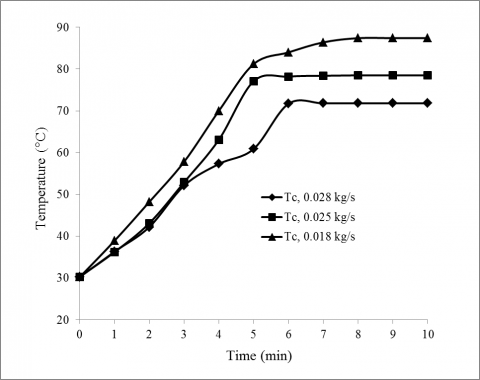Figure 8. Cold side temperature versus time for different air flow rates (hot side temperature: 130 °C)

## 9.pngFigure 9. Effect of air flow rate on temperature difference between the hot and cold sides and the TE modules (hot side temperature: 130°C)

The TE generator system conversion efficiency was calculated under hot and cold side temperatures as shown in Eq. (1). Figure 10 shows TE conversion efficiency and maximum power output at different cooling air flow rates; obviously, the conversion efficiency and maximum power output increased as air flow rate increased. Thus the power output and conversion efficiency increased from 3.7 to 9.3 W and 1.19 to 2.96 %, respectively, when the air flow rate increased from 0.018 to 0.028 kg/s. This represents a 148 % improvement in conversion efficiency. Consequently, a high air flow rate (0.028 kg/s) is recommended.

## 10.png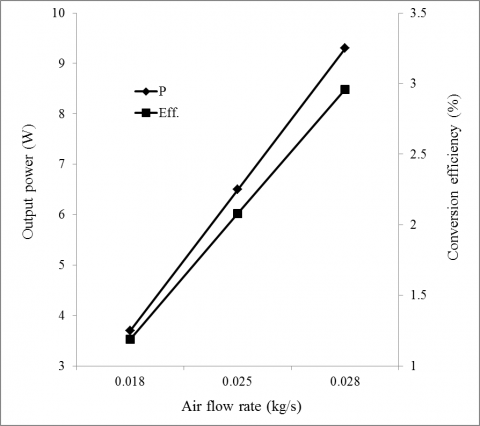Figure 10. Effect of air flow rate on output power and conversion efficiency (hot side temperature: 130°C)

4.2 Effect of hot side temperature

The valve controlling the LPG flow rate was adjusted to vary the hot side temperature. The influence of the hot side temperature on the performance of the TE modules is examined in Figure 11, where the air flow rate was fixed at 0.028 kg/s. It is apparent that the higher the hot side temperature, the larger the temperature difference. For the hot side temperatures of 80, 110, 130 °C, the temperature differences were 35.4, 45.3 and 60.9 °C, respectively, as shown in Figure 12.

## 11.png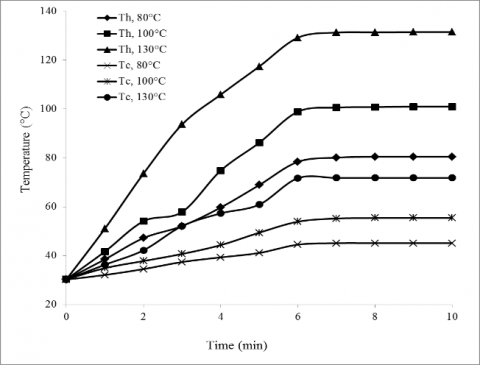Figure 11. Temperature profile of hot and cold sides of TE modules for different hot side temperature (air flow rate: 0.028 kg/s)

## 12.png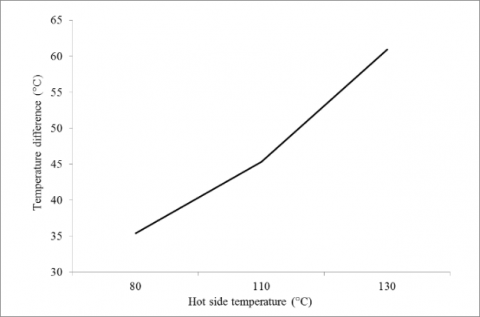Figure 12. Effect of hot side temperature on temperature difference between the hot and cold sides and the TE modules (air flow rate: 0.028 kg/s)

Corresponding to the preceding temperature differences, the open circuit voltage varied from 5.2 to 8.2 V and the maximum power output varied from 4 to 9.3 W, respectively (see Table 1). Of the three hot side temperatures evaluated, only the output voltage at the hot side temperature of 130 °C was enough to charge the battery and light LEDs. Therefore, the hot side temperature is suggested to be set at 130 °C.

Table 1. Variations of electric current, voltage and power output with hot side temperature difference (air flow rate: 0.028 kg/s)

 Hot side temperature (°C) Volt (V) I (A) P (W) 80 5.2 0.77 4.0 100 6.9 0.96 6.6 130 8.2 1.14 9.3

4.3 The effect of air flow direction

In this test setup, the fan was designed to pull air from the top of the heat sink or push air to the top of heat sink. Figure 13 shows the effect of air flow direction on the cold side of the TE modules. Obviously, when pushing air the cold side temperature was lower than pulling air by about 14.6 °C. Pushing the air caused turbulence at the base of the fins; whereas, when the fan was pulling the air little turbulence was created at the base of the heat sink . Turbulence tends to enhance heat transfer. Thus, when the pulling air was changed to pushing air, the temperature difference and power output increased from 45.6 to 61.5 °C and 4.5 W to 9.3 W, respectively as shown in Figure 14. Therefore, pushing air is recommended.

## 13.pngFigure 13. Cold side temperature versus time for different air flow direction

## 14.pngFigure 14. Effect of air flow direction on temperature difference between the hot and cold sides and the TE modules and power output

4.4 Theoretical model validation

Figure 15 shows a comparison of the experimental results and the theoretical predictions of the cold side temperature. The values are acceptable, with an error lower than 9 %. This difference is probably due to the fact that the convection heat transfer coefficient is supposed constant in the present model. In fact the convection heat transfer coefficient depends strongly on the temperature .

## 15.pngFigure 15. A comparison between experimental and theoretical cold side temperature as a function of hot side temperature (air flow rate: 0.028 kg/s)

When designing a TE generator for electrical generation purposes, information on the temperature difference between the hot and cold sides of the TE modules is important. A regression technique was used to curve fit for electrical power as a function of the temperature difference between the hot and cold sides of the TE modules as follows:

$\mathrm{T}=0.0015 \Delta \mathrm{T}^{2}+0.0673 \Delta \mathrm{T}$   (15)

The plot of experimental data and regression line for the output power and temperature difference is shown in Figure 16. The coefficient of determination of the regression model is 0.983.

## 16.png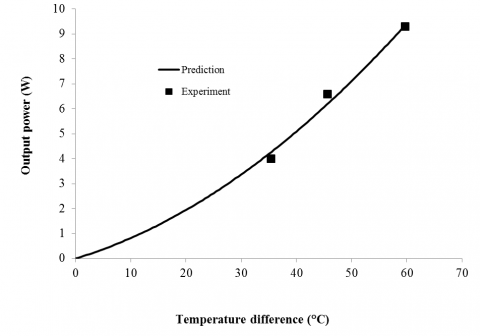Figure 16. A comparison between experimental data and regression line

4.5 Economic evaluation

To evaluate the economic viability, the payback period of the TE power generation system is determined. Measured in years (y), payback period is defined as investment of time required for the profit of an investment to equal the cost of the investment . The cost analysis of the TE generator system is evaluated and compared with the cost of energy supply (2 batteries size AA, 1.5 V) to a 2.7 W load (3 LEDs). The formula used here is calculated by .

$\mathrm{J}=\mathrm{B}\left[\frac{(1+\mathrm{i})^{\mathrm{y}}-1}{\mathrm{i}(1+\mathrm{i})^{\mathrm{y}}}\right]$        (16)

where J represents the initial investment cost of the TE generation system, B is the annual net undiscounted benefit, i is the interest rate. Interest rate of leading banks is supposed equal to 8 % for Thailand. In addition, fifteen years is considered the life of the TE system . The conditions for evaluating the economic analysis are summarized in Table 2.

Table 2. Conditions for economic evaluation

 Item Hot side temperature (°C) 130 Cooling air flowrate (kg/s) 0.028 Load (2.7 W) LEDs Fist cost (US$) 91.25 Operating cost - Lift cycle 15 Batteries (size AA, 1.5 Vⅹ 4) cost (US$/h) 0.18 Interest rate (%) 8

It was found that the payback period is decreased as the annual operating time increased. The payback period is about 1 year at the annual operating time of 550 hours as shown in Figure 17.

## 17.pngFigure 17. Payback period of the TE system (base on operating time)

5. Conclusions

The potential of TE-LPG cook stove for electrical power generation has been discussed in detail. An experiment has been carried out to characterize the electrical performance at various air cooling flow rates, hot side temperatures and fan orientations. All of the various parameters have an impact on the electrical performance. The results revealed that a maximum power output of 9.3 W for a temperature difference of 61.5 °C can be achieved from the TE generation system. This generated power was enough to charge a lead-acid battery that drove a cooling fan and light LEDs. A thermal model has been developed to predict the cold side temperature of the TE modules. The calculated results were in good agreement with the experimental results. This experimental work provides useful information to increases the effectiveness of combined TE power generation system with a cook stove. A simple economic analysis indicted that the payback period is 1 year, when compared with batteries supplying power to a 2.7 W load with an annual operating time of 550 hours.

Acknowledgement

The authors gratefully acknowledge the financial support provide by the Thailand Research Fund (Grant no. DPG5980004).

Nomenclature
 A area (m2) Dh hydraulic diameter (m) H height of fin (m) I output current of TE module (A) L length of TE side or heat sink (m) P output power of TE module (W) Pr Prandtl number Q heat transfer (W) R thermal resistance base on or electrical resistance of TE ($\Omega$) V output voltage of TE module (v) T ambient temperature (K) Z figure of merit of the TE materials (1/K) i Interest rate (%) k thermal conductance (WK-1) h heat transfer coefficient (W m-2K-1) n Number s pitch between fins (m) t thickness (m) y time (year) Greek symbols $\eta_{\mathrm{c}}$ carnot efficiency $\eta_{\mathrm{e}}$ conversion efficiency $\eta_{\mathrm{f}}$ fin efficiency Subscripts a ambient ai input of the air al aluminum ao output of the air b heat sink base c cold side temperatures of TE module (K) f fin h hot side temperatures of TE module (K) m average
References

 Makmool, U., Jugjai, S., Tia, S. (2007). Performance and analysis by particle image velocimetry (PIV) of cooker-top burners in Thailand. Energy, 32(10): 1986-1995. https://doi.org/10.1016/j.energy.2007.03.008

 Nuwayhid, R.Y., Rowe, D.M., Min, G. (2003). Low cost stove-top thermoelectric generator for regions with unreliable electricity supply. Renewable Energy, 28(2): 205-222. https://doi.org/10.1016/S0960-1481(02)00024-1

 Lertsatitthanakorn, C. (2007). Electrical performance and economic evaluation of combined biomass cook stove thermoelectric (BITE) generator. Bioresource Technology, 98(8): 1670-1674. https://doi.org/10.1016/j.biortech.2006.05.048

 Champier, D., Bedecarrats, J.P., Rivaletto, M., Strub, F. (2010). Thermoelectric power generation from biomass cook stoves. Energy, 35(2): 935-942. https://doi.org/10.1016/j.energy.2009.07.015

 Champier, D., Bedecarrats, J.P., Kousksou, T., Rivaletto, M., Strub, F., Pignolet, P. (2011). Study of a TE (thermoelectric) generator incorporated in a multifunction wood stove. Energy, 36(3): 1518-1526. https://doi.org/10.1016/j.energy.2011.01.012

 O’Shaughnessy, S.M., Deasy, M.J., Kinslla, C.E. (2012). Small scale electricity generation from a portable biomass cookstove: Protoype design and preliminary results. Applied Energy, 102: 374-385. https://doi.org/10.1016/j.apenergy.2012.07.032

 Montecucco, A., Siviter, J., Knox, A.R. (2017). Combined heat and power system for stoves with thermoelectric generators. Applied Energy, 185: 1336-1342. https://doi.org/10.1016/j.apenergy.2015.10.132

 Najjar, S.H.Y., Kseibi, M. (2017). Evaluation of experimental JUST thermoelectric stove for electricity-Deprived regions. Renewable and Sustainable Energy Reviews, 69: 854-861. https://doi.org/10.1016/j.rser.2016.07.041

 Li, G.N., Zhang, S., Zheng, Y.Q., Zhu, L.Y., Guo, W.W. (2018). Experimental study on a stove-powered thermoelectric generator (STEG) with self starting fan cooling. Renewable Energy, 121: 502-512. https://doi.org/10.1016/j.renene.2018.01.075

 Obernberger, I., Weib, G., Kossl, M. (2018). Development of a new micro CHP pellet stove technology. Biomass and Bioenergy, 116: 198-204. https://doi.org/10.1016/j.biombioe.2018.06.020

 Lv, H., Zheng, Y., Hu, J., Li, J. (2018). Compact water-cooled thermoelectric generator (TEG) based on a portable gas stove. Energies, 11(9): 2231-2250. https://doi.org/10.3390/en11092231

 Meghdir, A., Benabdallah, T., Dellil, Z.E.A. (2019). Impact of geometry of electronic components on cooling improvement. International Journal of Heat and Technology, 37(1): 167-178. https://doi.org/10.18280/ijht.370121

 Cengel, Y.A. (2003). Heat Transfer: A Practical Approach. 2nd ed., McGraw-Hill, New York.

 Boonyasri, M., Jamradloedluk, J., Lertsatitthanakorn, C., Therdyothin, A., Soponronnarit, S. (2017). Increasing the efficiency of a thermoelectric generator using an evaporative cooling system. Journal of Electronic Materials, 46(5): 3043-3046. https://doi.org/10.1007/s11664-016-5142-9

 Chang, Y.W., Chang, C.C., Ke, M.T., Chen, S.L. (2009). Thermoelectric air-cooling module for electronic devices. Applied Thermal Engineering, 29(13): 2731-2737. https://doi.org/10.1016/j.applthermaleng.2009.01.004

 Banakar, A., Motevali, A., Emad, M., Ghobadian, B. (2017). Co-generation of heat and power in a thermoelectric system equipped with Fresnel lens collectors using active and passive cooling techniques. Renewable Energy, 112: 268-279. https://doi.org/10.1016/j.renene.2017.05.052

 Ritzer, M.T., Lau, G.P. (2000). The effect of fan orientation on heat sink performance. The 19th International Conference on Thermoelectrics, Cardiff, UK, pp. 333-338.

 Chen, H.T., Lai, S.T., Haung, L.Y. (2013). Investigation of heat transfer characteristics in plate-fin heat sink. Applied Thermal Engineering, 50(1): 352-360. https://doi.org/10.1016/j.applthermaleng.2012.08.040

 Newnan, D.G. (1980). Engineering economic analysis. Engineering Press Inc., California.

 Blank, L.T., Tarquin, A. (1998). Engineering economy. McGraw-Hill, Singapore.